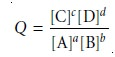Home | | Modern Analytical Chemistry | Thermodynamics and Equilibrium Chemistry

# Thermodynamics and Equilibrium Chemistry

Thermodynamics is the study of thermal, electrical, chemical, and mechanical forms of energy.

Thermodynamics and Equilibrium Chemistry

Thermodynamics is the study of thermal, electrical, chemical, and mechanical forms of energy. The study of thermodynamics crosses many disciplines, including physics, engineering, and chemistry. Of the various branches of thermodynamics the most important to chemistry is the study of the changes in energy occurring during a chemical reaction.

Consider, for example, the general equilibrium reaction shown in equation 6.1, involving the solutes A, B, C, and D, with stoichiometric coefficients a, b, c, and d.

aA+ bB < = = = = > cC+ dD

By convention, species to the left of the arrows are called reactants, and those on the right side of the arrows are called products. As Berthollet discovered, writing a reac- tion in this fashion does not guarantee that the reaction of A and B to produce C and D is favorable. Depending on initial conditions, the reaction may move to the left, to the right, or be in a state of equilibrium. Understanding the factors that determine the final position of a reaction is one of the goals of chemical thermodynamics.

Chemical systems spontaneously react in a fashion that lowers their overall free energy. At a constant temperature and pressure, typical of many bench-top chemi- cal reactions, the free energy of a chemical reaction is given by the GibbÔÇÖs free en- ergy function

ÔêåG = ÔêåH ÔÇô T ÔêåS

where T is the temperature in kelvins, and ÔêåG, ÔêåH, and ÔêåS are the differences in the GibbÔÇÖs free energy, the enthalpy, and the entropy between the products and reactants.

Enthalpy is a measure of the net flow of energy, as heat, during a chemical re- action. Reactions in which heat is produced have a negative ÔêåH and are called exothermic. Endothermic reactions absorb heat from their surroundings and have a positive ÔêåH. Entropy is a measure of randomness, or disorder. The entropy of an individual species is always positive and tends to be larger for gases than for solids and for more complex rather than simpler molecules. Reactions that result in a large number of simple, gaseous products usually have a positive ÔêåS.

The sign of ÔêåG can be used to predict the direction in which a reaction moves to reach its equilibrium position. A reaction is always thermodynamically favored when enthalpy decreases and entropy increases. Substituting the inequalities ÔêåH <0 and ÔêåS > 0 into equation 6.2 shows that ÔêåG is negative when a reaction is thermo- dynamically favored. When ÔêåG is positive, the reaction is unfavorable as written (although the reverse reaction is favorable). Systems at equilibrium have a ÔêåG of zero.

As a system moves from a nonequilibrium to an equilibrium position, ÔêåG must change from its initial value to zero. At the same time, the species involved in the reaction undergo a change in their concentrations. The GibbÔÇÖs free energy, there- fore, must be a function of the concentrations of reactants and products.

As shown in equation 6.3, the GibbÔÇÖs free energy can be divided into two terms.

ÔêåG = ÔêåG┬░+ RT ln Q         ÔÇªÔÇªÔÇªÔÇª6.3

The first term, ÔêåG┬░, is the change in GibbÔÇÖs free energy under standard-state condi- tions; defined as a temperature of 298 K, all gases with partial pressures of 1 atm, all solids and liquids pure, and all solutes present with 1 M concentrations. The second term, which includes the reaction quotient, Q, accounts for nonstandard-state pres- sures or concentrations. For reaction 6.1 the reaction quotient iswhere the terms in brackets are the molar concentrations of the solutes. Note that the reaction quotient is defined such that the concentrations of products are placed in the numerator, and the concentrations of reactants are placed in the denominator. In addition, each concentration term is raised to a power equal to its stoichiometric coefficient in the balanced chemical reaction. Partial pressures are substituted for concentrations when the reactant or product is a gas. The concentrations of pure solids and pure liquids do not change during a chemical reaction and are excluded from the reaction quotient.

At equilibrium the GibbÔÇÖs free energy is zero, and equation 6.3 simplifies to

ÔêåG┬░= ÔÇôRT ln K

where K is an equilibrium constant that defines the reactionÔÇÖs equilibrium posi- tion. The equilibrium constant is just the numerical value obtained when substitut- ing the concentrations of reactants and products at equilibrium into equation 6.4; thus,where the subscript ÔÇ£eqÔÇØ indicates a concentration at equilibrium. Although the subscript ÔÇ£eqÔÇØ is usually omitted, it is important to remember that the value of K is determined by the concentrations of solutes at equilibrium.

As written, equation 6.5 is a limiting law that applies only to infinitely dilute solutions, in which the chemical behavior of any species in the system is unaffected by all other species.

Study Material, Lecturing Notes, Assignment, Reference, Wiki description explanation, brief detail
Modern Analytical Chemistry: Equilibrium Chemistry : Thermodynamics and Equilibrium Chemistry |